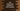# Java Arrays deepEquals method explanation with example## java.util.Arrays.deepEquals method explanation with examples:

In this post, we will learn how deepEquals method of java.util.Arrays class works. This method is different from the equals method and we can use it with nested arrays.

Let’s take a look at its definition.

### Definition of deepEquals:

This method is defined as like below:

``public static boolean deepEquals(Object[] firstArray, Object[] secondArray)``

This method takes two arrays as the parameters and returns one boolean value. It returns true if both arrays are deeply equal.

Two arrays are called deeply equal if:

• Both arrays are null.
• If they refer to arrays that hold the same number of elements and all pairs of these elements of these arrays are deeply equal.

Two possibly null elements first and second are deeply equal, if any of these is true:

• first == second
• If first.equals(second) is true.
• first and second are arrays of the same primitive type and Arrays.equal(first, second) returns true.
• first and second are arrays of object reference type and Arrays.deepEqual(first, second) returns true.

It permits null elements at any depth.

### Example with two-dimensional arrays:

Let’s try deepEquals with 2-D arrays:

``````import java.util.Arrays;

class Main {
public static void main(String[] args) {
int[][] firstArray = {{1, 2, 3, 4, 5}, {1, 2, 3}};
int[][] secondArray = {{1, 2, 3, 4, 5}, {1, 2, 3}};
int[][] thirdArray = {{1, 3, 4, 5}, {1, 2, 3}};

System.out.println("Arrays.deepEquals(firstArray, secondArray) = " + Arrays.deepEquals(firstArray, secondArray));
System.out.println("Arrays.deepEquals(firstArray, thirdArray) = " + Arrays.deepEquals(firstArray, thirdArray));
}
}``````

It will print:

``````Arrays.deepEquals(firstArray, secondArray) = true
Arrays.deepEquals(firstArray, thirdArray) = false``````

### Example with object arrays:

We can also use deepEquals with an array of objects. For example:

``````import java.util.Arrays;

class Student {
String name;
int age;

Student(String name, int age) {
this.name = name;
this.age = age;
}
}

class Main {
public static void main(String[] args) {
Student alex = new Student("Alex", 10);
Student bob = new Student("Bob", 20);

Student[] firstArray = {alex, bob};
Student[] secondArray = {alex, bob};
Student[] thirdArray = {new Student("Alex", 10), new Student("Bob", 20)};

System.out.println("Arrays.deepEquals(firstArray, secondArray) = " + Arrays.deepEquals(firstArray, secondArray));
System.out.println("Arrays.deepEquals(firstArray, thirdArray) = " + Arrays.deepEquals(firstArray, thirdArray));
}
}``````

In this example, we are using an array of Student class objects.

It will print:

``````Arrays.deepEquals(firstArray, secondArray) = true
Arrays.deepEquals(firstArray, thirdArray) = false``````

Even though both firstArray and thirdArray hold objects with the same contents, but they are not referring to the same instances of the objects. So deepEquals returns false.